# Precalculus : Find the Product of Complex Numbers

## Example Questions

### Example Question #1 : Polar Coordinates And Complex Numbers

Find the value of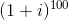,wherethe complex number is given by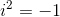.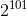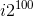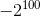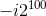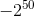Explanation:

We note that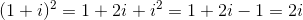by FOILing.

We also know that: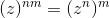We have by using the above rule: n=2 , m=50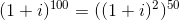Since we know that,We have then: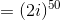Since we know that: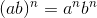, we use a=2 ,b=i

We have then: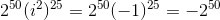### Example Question #1 : Find The Product Of Complex Numbers

Compute the following sum: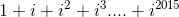. Rememberis the complex number satisfying.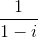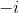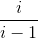Explanation:

Note that this is a geometric series.

Therefore we have: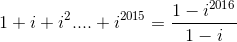Note that,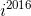=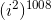and sincewe have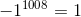.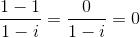this shows that the sum is 0.

### Example Question #1 : Polar Coordinates And Complex Numbers

Find the following product.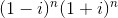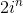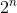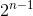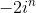Explanation:

Note that by FOILing the two binomials we get the following: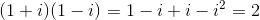Therefore,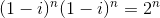### Example Question #1 : Polar Coordinates And Complex Numbers

Compute the magnitude of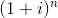.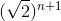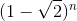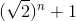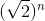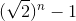Explanation:

We have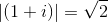We know that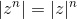Thus this gives us,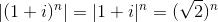.

### Example Question #2 : Products And Quotients Of Complex Numbers In Polar Form

Evaluate: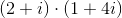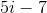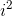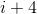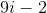Explanation:

To evaluate this problem we need to FOIL the binomials.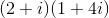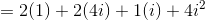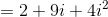Now recall thatThus,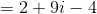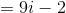### Example Question #1 : Find The Product Of Complex Numbers

Find the product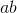, if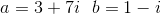.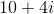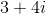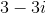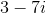Explanation:

To find the product, FOIL the complex numbers. FOIL stands for the multiplication of the Firsts, Outers, Inners, and Lasts.

Using this method we get the following,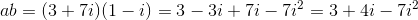and because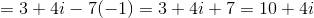.

### Example Question #1 : Polar Coordinates And Complex Numbers

Simplify: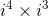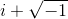Explanation:

The expressioncan be rewritten as: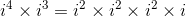Since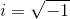, the value of.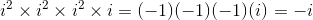The correct answer is:### Example Question #1 : Products And Quotients Of Complex Numbers In Polar Form

Find the product of the two complex numbers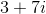and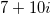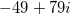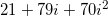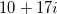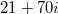Explanation:

The product is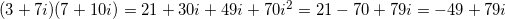### All Precalculus Resources